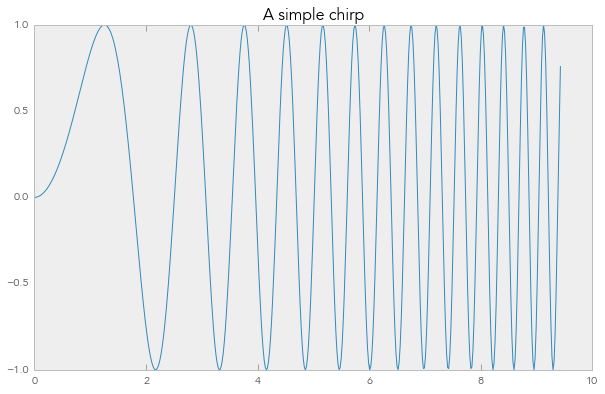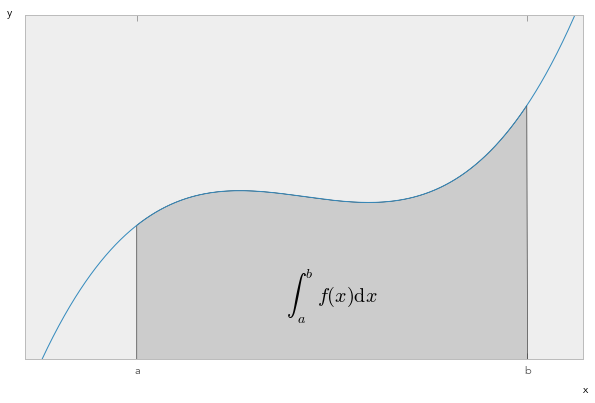Plotting with Matplotlib¶

IPython works with the Matplotlib plotting library, which integrates Matplotlib with IPython's display system and event loop handling.

matplotlib mode¶

To make plots using Matplotlib, you must first enable IPython's matplotlib mode.

To do this, run the %matplotlib magic command to enable plotting in the current Notebook.

This magic takes an optional argument that specifies which Matplotlib backend should be used. Most of the time, in the Notebook, you will want to use the inline backend, which will embed plots inside the Notebook:

In :
%matplotlib inline

You can also use Matplotlib GUI backends in the Notebook, such as the Qt backend (%matplotlib qt). This will use Matplotlib's interactive Qt UI in a floating window to the side of your browser. Of course, this only works if your browser is running on the same system as the Notebook Server. You can always call the display function to paste figures into the Notebook document.

Making a simple plot¶

With matplotlib enabled, plotting should just work.

In :
import matplotlib.pyplot as plt
import numpy as np
In :
x = np.linspace(0, 3*np.pi, 500)
plt.plot(x, np.sin(x**2))
plt.title('A simple chirp');These images can be resized by dragging the handle in the lower right corner. Double clicking will return them to their original size.

One thing to be aware of is that by default, the Figure object is cleared at the end of each cell, so you will need to issue all plotting commands for a single figure in a single cell.

IPython's %load magic can be used to load any Matplotlib demo by its URL:

In :
In :
#!/usr/bin/env python

# implement the example graphs/integral from pyx
from pylab import *
from matplotlib.patches import Polygon

def func(x):
return (x-3)*(x-5)*(x-7)+85

ax = subplot(111)

a, b = 2, 9 # integral area
x = arange(0, 10, 0.01)
y = func(x)
plot(x, y, linewidth=1)

ix = arange(a, b, 0.01)
iy = func(ix)
verts = [(a,0)] + list(zip(ix,iy)) + [(b,0)]
poly = Polygon(verts, facecolor='0.8', edgecolor='k')
r"$\int_a^b f(x)\mathrm{d}x$", horizontalalignment='center',